# 图像处理库 手机端可用

## 图像处理作业5——SIFT算法与全景图像生成

2020-05-04 15:46:16 qq_42662568 阅读数 1545
• ###### Linux系统目录及必备命令学习

通过本课程的学习，大家可以从懵懂到熟悉，从熟悉到熟练，能够在企业中熟练运用，同时在学习的过程中有任何不明白的地方，都可以向我咨询，我会积极帮助大家解决问题。

217人学习 吴光科
免费试看IDE：Jupyter notebook

## 1. 实验思路

（1）尝试采用SIFT特征描述子提取特征；

（2）尝试特征匹配；

（3）找到变换矩阵，变换图像；

（4）拼接融合图像。

## 2. 实验原理

### SIFT算法简介

SIFT (Scale-invariant feature transform)尺度不变特征转换即是一种计算机视觉的算法。它用来侦测与描述影像中的局部性特征，它在空间尺度中寻找极值点，并提取出其位置、尺度、旋转不变量，此算法由 David Lowe在1999年所发表，2004年完善总结。

SIFT算法的实质是在不同的尺度空间上查找关键点(特征点)，并计算出关键点的方向。SIFT所查找到的关键点是一些十分突出，不会因光照，仿射变换和噪音等因素而变化的点，如角点、边缘点、暗区的亮点及亮区的暗点等。

SIFT算法的特点有：

1. SIFT特征是图像的局部特征，其对旋转、尺度缩放、亮度变化保持不变性，对视角变化、仿射变换、噪声也保持一定程度的稳定性；

2. 独特性（Distinctiveness）好，信息量丰富，适用于在海量特征数据库中进行快速、准确的匹配；

3. 多量性，即使少数的几个物体也可以产生大量的SIFT特征向量；

4. 高速性，经优化的SIFT匹配算法甚至可以达到实时的要求；

5. 可扩展性，可以很方便的与其他形式的特征向量进行联合。

### 算法流程SIFT算法实现物体识别主要有三大工序，1、提取关键点；2、对关键点附加详细的信息（局部特征）也就是所谓的描述器；3、通过两方特征点（附带上特征向量的关键点）的两两比较找出相互匹配的若干对特征点，也就建立了景物间的对应关系。

### SIFT算法操作步骤

#### 1. 关键点检测

##### 1.2 什么是尺度空间（scale space）？

$G(x_i,y_i,\sigma)=\frac{1}{2\pi\sigma^2}exp(-\frac{(x-x_i)^2=(y-y_i)^2}{2\sigma^2})$

$L(x,y,\sigma)=G(x,y,\sigma)*I(x,y)$

##### 1.3 高斯模糊

$G(r)=\frac{1}{2\pi\sigma^2}exp(-\frac{r^2}{2\sigma^2})$

$r$为模糊半径，$r=\sqrt{x^2+y^2}$

##### 1.4 高斯金字塔$\sigma$——尺度空间坐标；s——sub-level层坐标；$\sigma_0$——初始尺度；$S$——每组层数（一般为3~5层）。

##### 1.5 差分高斯金字塔##### 1.6 极值点检测##### 1.7 关键点精确定位

$\left.\frac{1}{2}\left([\begin{array}{ccccccc}x & y & \sigma\end{array}\right]-\left[\begin{array}{ccc}x_{0} & y_{0} & \sigma_{0}\end{array}\right]\right)\left[\begin{array}{ccc}\frac{\partial^{2} f}{\partial x \partial x} & \frac{\partial^{2} f}{\partial x \partial y} & \frac{\partial^{2} f}{\partial x \partial \sigma} \\ \frac{\partial^{2} f}{\partial x \partial y} & \frac{\partial^{2} f}{\partial y \partial y} & \frac{\partial^{2} \pi}{\partial y \partial \sigma} \\ \frac{\partial^{2} f}{\partial x \partial \sigma} & \frac{\partial^{2} f}{\partial y \partial \sigma} & \frac{\partial^{2} f}{\partial \sigma \partial \sigma}\end{array}\right]\left(\left[\begin{array}{l} x \\ y \\ \sigma \end{array}\right]-\left[\begin{array}{l} x_{0} \\ y_{0} \\ \sigma_{0} \end{array}\right]\right)$

$\frac{\partial f}{\partial x}=\frac{f(i, j+1)-f(i, j-1)}{2 h}, \quad \frac{\partial f}{\partial y}=\frac{f(i+1, j)-f(i-1, j)}{2 h}$

$\frac{\partial^{2} f}{\partial x^{2}}=\frac{f(i, j+1)+f(i, j-1)-2 f(i, j)}{h^{2}}, \quad \frac{\partial^{2} f}{\partial y^{2}}=\frac{f(i+1, j)+f(i-1, j)-2 j}{h^{2}}$

$\frac{\partial^{2} f}{\partial x \partial y}=\frac{f(i-1, j-1)+f(i+1, j+1)-f(i-1, j+1)-f(i+1, j-1)}{4 h^{2}}$

$D(X)=D+\frac{\partial D^{T}}{\partial X} X+\frac{1}{2} X^{T} \frac{\partial^{2} D}{\partial X^{2}} X$，其中，X求导并让方程等于0，可得极值点的偏移量为$\hat{X}=-\frac{\partial^{2} D^{-1}}{\partial X^{2}} \frac{\partial D}{\partial X}$，对应极值点，方程的值为$D(\hat{X})=D+\frac{1}{2} \frac{\partial D^{T}}{\partial X} \hat{X}$

#### 2. 关键点描述

##### 2.1 关键点方向匹配

（1）梯度计算（2）梯度直方图

1. 直方图以每10度方向为一个柱，共36个柱，柱所代表的的方向为像素点梯度方向，柱的长短代表了梯度幅值。
2. 根据Lowe的建议，直方图1统计半径采用$3*1.5*\sigma$
3. 在直方图统计时每相邻三个像素点采用高斯加权，模板采用$[0.25,0.5,0.25]$，并连续加权两次。（3）特征点主方向的确定

$H(i)=\frac{h(i-2)+h(i+2)}{16}+\frac{4 \times(h(i-1)+h(i+1))}{16}+\frac{6 \times h(i)}{16}$

（4）梯度直方图抛物线插值$h(t)'=0$$t_max=-b/(2a)$。现在把这三个插值点代入方程可得：

$\left.\begin{array}{l}\mathrm{h}(-1)=\mathrm{a}-\mathrm{b}+\mathrm{c} \\ \mathrm{h}(0)=\mathrm{c} \\ \mathrm{h}(1)=\mathrm{a}+\mathrm{b}+\mathrm{c}\end{array}\right\}$——>$\left\{\begin{array}{l}-\mathrm{a}=[\mathrm{h}(1)+\mathrm{h}(-1)] / 2-\mathrm{h}(0) \\ \mathrm{b}=[\mathrm{h}(1)-\mathrm{h}(-1)] / 2 \\ \mathrm{c}=\mathrm{h}(0)\end{array}\right.$

$\mathbf{i}^{\prime}=\mathbf{i}+\frac{h(i-1)-h(i+1)}{2[h(i-1)+h(i+1)-2 h(i)]}$（小柱子在直方图中的索引号）。

##### 2.2 生成描述符

（1）确定计算描述子所需的区域

radius$=\frac{3 \sigma_{\text {oct}} \times \sqrt{2} \times(d+1)+1}{2}$$\sigma_{oct}$是关键点所在组（octave）的组内尺度，$d=4$（2）将坐标移至关键点主方向（3）梯度直方图的生成（4）三线性插值（5）描述子生成过程### 单应矩阵（Homography）

1. 首先检测两边图像的角点
2. 在角点之间应用方差归一化相关，收集相关性足够高的对，形成一组候选匹配。
3. 选择四个点，计算H
4. 选择与单应性一致的配对。如果对于某些阈值:Dist(Hp、q) <ε，则点对(p, q)被认为与单应性H一致
5. 重复34步，直到足够多的点对满足H
6. 使用所有满足条件的点对，通过公式重新计算H

RANSAC（Random Sample Consensus，随机抽样一致）是一种鲁棒性的参数估计方法。它的实质就是一个反复测试、不断迭代的过程。### 图像变形和融合

（1）图像变形

1. 首先计算每个输入图像的变形图像坐标范围，得到输出图像大小，可以很容易地通过映射每个源图像的四个角并且计算坐标(x,y)的最小值和最大值确定输出图像的大小。最后，需要计算指定参考图像原点相对于输出全景图的偏移量的偏移量x_offset和偏移量y_offset。
2. 下一步是使用上面所述的反向变形，将每个输入图像的像素映射到参考图像定义的平面上，分别执行点的正向变形和反向变形。

（2）图像融合

$[(\alpha_1R,\alpha_1G,\alpha_1B,\alpha_1)+(\alpha_2R,\alpha_2G,\alpha_2B,\alpha_2)]/(\alpha_1+\alpha_2)$

## 3 代码实现

#################
#Author：Tian YJ#
#图像拼接实现全景图#
#################

# 导入基本库文件
import numpy as np
from numpy import *
from numpy.linalg import det, lstsq, norm # 线性代数模块
import cv2
import matplotlib.pyplot as plt
from functools import cmp_to_key # 接受两个参数，将两个参数做处理

# 加上这两行可以一次性输出多个变量而不用print
from IPython.core.interactiveshell import InteractiveShell
InteractiveShell.ast_node_interactivity = "all"
# 设置容忍度
float_tolerance = 1e-7

%matplotlib inline

##################
#设置路径、读取图片#
##################
# 设置路径
path = 'C:\\Users\\86187\\Desktop\\image\\'
# 读取待拼接图片（灰度图）
# img1为左图，img2位右图
img1 = cv2.imread(path + 'left.jpg', 0)
img2 = cv2.imread(path + 'right.jpg', 0)

# 原始图片展示
plt.figure(figsize=(25,10))
plt.subplot(1,2,1)
plt.imshow(img1.astype(np.uint8), cmap="gray")
plt.subplot(1,2,2)
plt.imshow(img2.astype(np.uint8), cmap="gray")
plt.show()#############################
#对图像进行倍数放大（双线性插值）#
#############################
def resize(img, ratio=2.):
"""
img: 待处理图片
ratio: 放大倍数
"""
# 目标图像尺寸
new_shape = [int(img.shape * ratio), int(img.shape * ratio)]
result = np.zeros((new_shape))  # 目标图像初始化
# 遍历新的图像坐标
for h in range(new_shape):
for w in range(new_shape):
# 对应的原图像上的点(向下取整，也就是左上点的位置)
h0 = int(np.floor(h / ratio))
w0 = int(np.floor(w / ratio))
# 新图像的坐标/放缩比例 - 原图像坐标点 = 距离
dx = h / ratio - h0
dy = w / ratio - w0
# 防止溢出
h1 = h0 + 1 if h0 < img.shape - 1 else h0
w1 = w0 + 1 if w0 < img.shape - 1 else w0
# 进行插值计算
result[h, w] = (1 - dx) * (1 - dy) * img[h0, w0] + dx * (
1 - dy) * img[h1, w0] + (
1 - dx) * dy * img[h0, w1] + dx * dy * img[h1, w1]
result = result.astype(np.uint8)
return result

##################
#对图像进行边缘填充#
##################
"""
img: 待处理图片
"""

# 获取图片尺寸
H, W = img.shape

# 先填充行
# 再填充列
# 进行拼接
img = np.vstack((rows, img, rows))  # 上下拼接
img = np.hstack((cols, img, cols))  # 左右拼接

# 第一步，上下边框对称取值
img[0, :] = img[2, :]
img[-1, :] = img[-3, :]
# 第二步，左右边框对称取值
img[:, 0] = img[:, 2]
img[:, -1] = img[:, -3]
# 第三步，四个顶点对称
img[0, 0] = img[0, 2]
img[-1, 0] = img[-1, 2]
img[0, -1] = img[0, -3]
img[-1, -1] = img[-1, -3]

return img

##############
#设置滤波器系数#
##############
def Kernel(K_sigma, K_size):
"""
K_sigma: 模糊度
K_size: 滤波器即卷积核尺寸
"""

# 对滤波器进行初始化0
K = np.zeros((K_size, K_size), dtype=np.float)

# 代入公式求高斯滤波器系数，并填入矩阵
K[y + pad, x + pad] = np.exp(-(x**2 + y**2) / (2 * (K_sigma**2)))

K /= K.sum()  # 进行归一化

return K

#############
#进行高斯滤波#
#############
def gaussFilter(img, K_size=5, K_sigma=1.6):
"""
img: 需要处理图像
K_size: 滤波器尺寸
K_sigma: 模糊度
"""

# 获取图片尺寸
H, W = img.shape

# 滤波器矩阵
K = Kernel(K_sigma, K_size)

## 进行滤波
out = img.copy()
for h in range(H):
for w in range(W):
out[pad + h, pad + w] = np.sum(K * out[h:h + K_size, w:w + K_size])
# 截取像素合理值
out = out / out.max() * 255
return out

##################
#生成金字塔基础图像#
##################
def generateBaseImage(image, sigma, assumed_blur):
"""
将输入图像放大一倍并应用高斯模糊，以生成图像金字塔的基础图像
image: 待处理图片
sigma: 目标模糊度
assumed_blur: 假设模糊度
"""
# 进行2倍放大
image = resize(image, ratio=2.0)
# 对图像应用多个连续的高斯模糊效果与应用单个较大的高斯模糊效果相同
sigma_diff = np.sqrt(max((sigma**2) - ((2 * assumed_blur)**2), 0.01))

return gaussFilter(image, K_size=5, K_sigma=sigma_diff)

# 尝试一下
base_image = generateBaseImage(img1, 1.6, 0.5)
# cv2.imshow('result', base_image)
# cv2.imshow('begin', img1)
# cv2.waitKey(0)

####################################
#计算可以将图像重复减半直到变得很小的次数#
####################################
def computeNumberOfOctaves(image_shape):
"""
image_shape: 图像尺寸
"""
return int(round(np.log(min(image_shape)) / np.log(2) - 1))

####################################
#为特定图层中的每个图像创建一个模糊度列表#
####################################
def generateGaussianKernels(sigma, num_intervals):
"""
sigma: 模糊度
num_intervals: 能进行极值点检测的图层数
高斯金字塔每组有num_intervals+1+2层
"""
# 高斯金字塔每组层数
num_images_per_octave = num_intervals + 3
# 高斯模糊度系数
k = 2**(1. / num_intervals)
# 高斯模糊度列表初始化为0
gaussian_kernels = np.zeros(num_images_per_octave)
# 第一个高斯模糊度
gaussian_kernels = sigma

# 第0层在升采样时已进行高斯模糊，故从第1层开始
for image_index in range(1, num_images_per_octave):
sigma_previous = (k**(image_index - 1)) * sigma
sigma_total = k * sigma_previous
gaussian_kernels[image_index] = np.sqrt(sigma_total**2 -
sigma_previous**2)
return gaussian_kernels

#####################
#生成尺度空间高斯金字塔#
#####################
def generateGaussianImages(image, num_octaves, gaussian_kernels):
"""
image: 输入基图像
num_octaves: 尺度金字塔组数
gaussian_kernels: 每一组的高斯模糊度列表
"""
# 总的高斯金字塔列表
gaussian_images = []

for octave_index in range(num_octaves):
# 每一组的高斯金字塔列表
gaussian_images_in_octave = []
gaussian_images_in_octave.append(image)  # 第一个图像已经滤波
for gaussian_kernel in gaussian_kernels[1:]:
# 进行高斯滤波
image = gaussFilter(image, K_size=5, K_sigma=gaussian_kernel)
gaussian_images_in_octave.append(image)
gaussian_images.append(gaussian_images_in_octave)
# 将上一组的倒数第三层作为下一组的基图像
octave_base = gaussian_images_in_octave[-3]  # 倒数第三层
image = octave_base[::2, ::2]  # 下采样
return array(gaussian_images)

# 打印高斯模糊度列表
gaussian_kernels = generateGaussianKernels(1.6, 3)
print(gaussian_kernels)
# 显示高斯金字塔图像
gaussian_images = generateGaussianImages(base_image, 8, gaussian_kernels)

for k in range(len(gaussian_images)):
plt.figure(figsize=(25, 10))
for i in range(len(gaussian_images[k])):
plt.subplot(1, len(gaussian_images[k]), i + 1)
plt.imshow(gaussian_images[k][i].astype(np.uint8), cmap="gray")
plt.show()


[1.6 1.2262735 1.54500779 1.94658784 2.452547 3.09001559]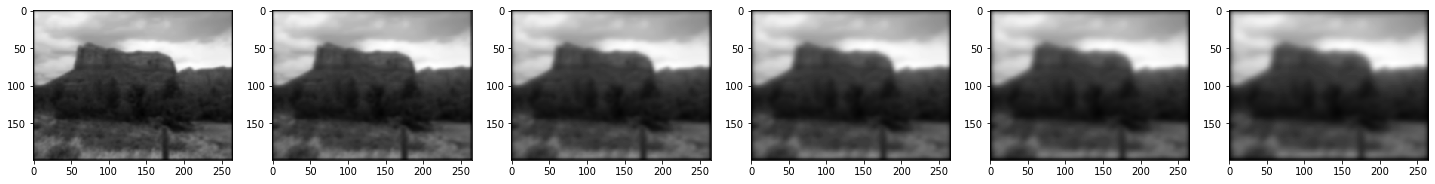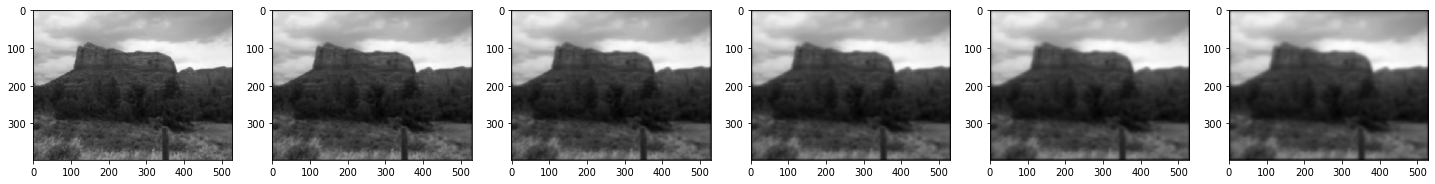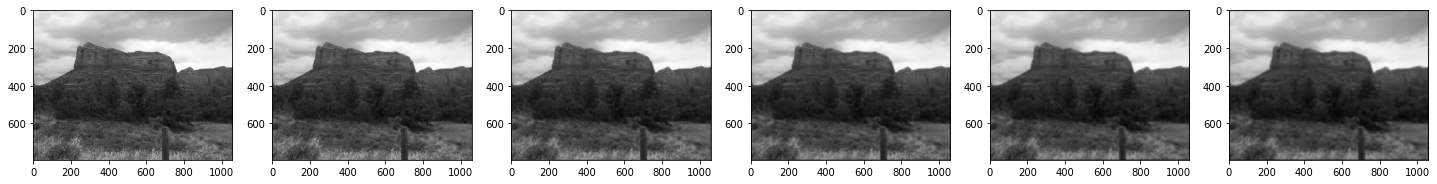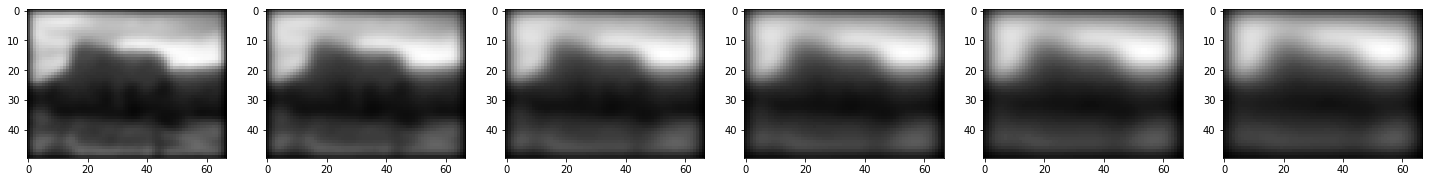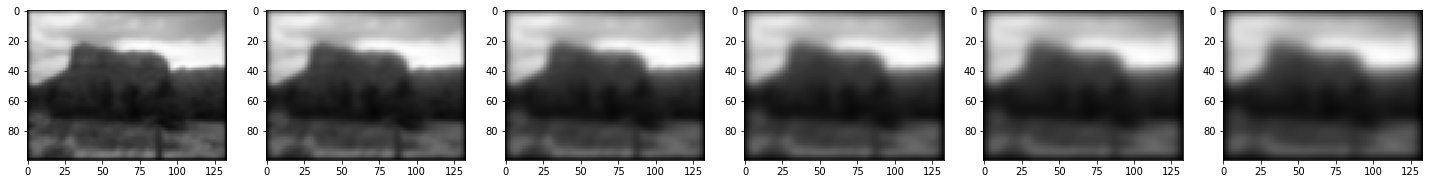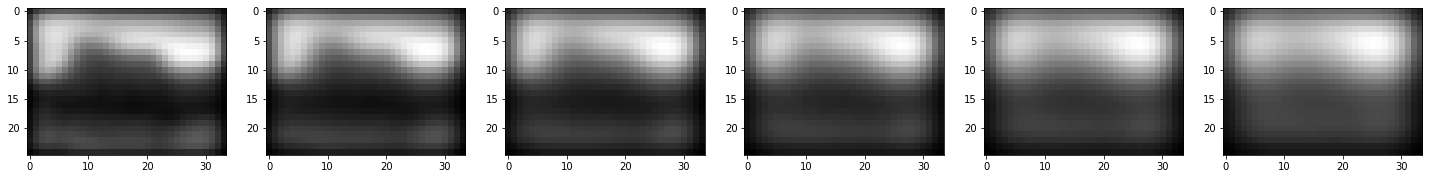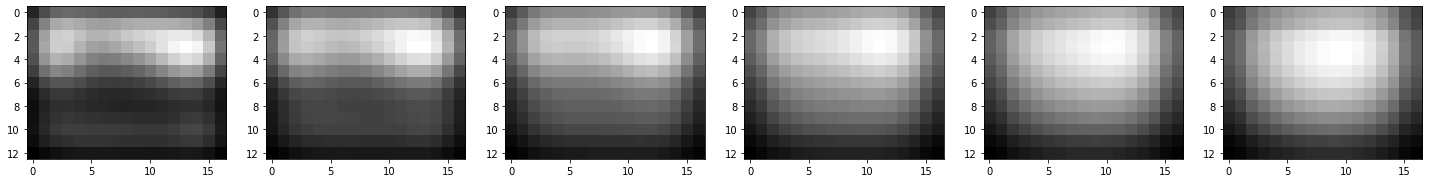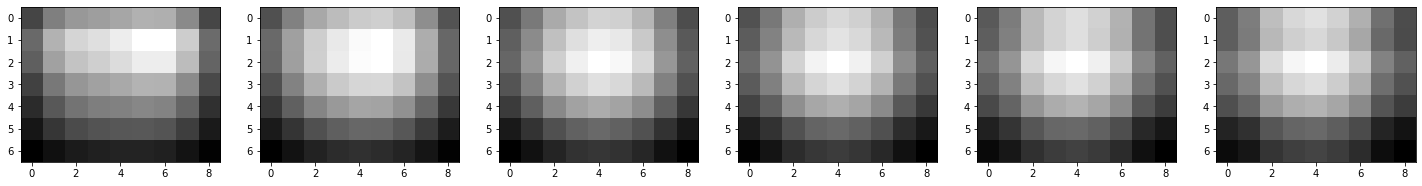#################
#生成高斯差分金字塔#
#################
def generateDoGImages(gaussian_images):
"""
gaussian_images: 传入高斯金字塔组
"""
# 总的差分金字塔列表
dog_images = []

for gaussian_images_in_octave in gaussian_images:
# 每一组高斯差分金字塔列表
dog_images_in_octave = []
# 两两进行差分运算
for first_image, second_image in zip(gaussian_images_in_octave,
gaussian_images_in_octave[1:]):
dog_images_in_octave.append(cv2.subtract(
second_image, first_image))  # 普通的减法不行，因为图像是无符号整数
dog_images.append(dog_images_in_octave)
return array(dog_images)

# 显示差分金字塔图像
dog_images = generateDoGImages(gaussian_images)
for k in range(len(dog_images)):
plt.figure(figsize=(25, 10))
for i in range(len(dog_images[k])):
plt.subplot(1, len(dog_images[k]), i + 1)
plt.imshow(dog_images[k][i].astype(np.uint8), cmap="gray")
plt.show()####################
#查找极值点的像素位置#
####################
def findScaleSpaceExtrema(gaussian_images,
dog_images,
num_intervals,
sigma,
image_border_width,
contrast_threshold=0.04):
"""
gaussian_images: 高斯金字塔组
dog_images: 差分金字塔组
num_intervals：每一组极值点检测层数
sigma：模糊度
image_border_width：靠近图像边缘5个像素的区域不做检测
contrast_threshold：对比度阈值
"""
# 阈值化，不保留低于阈值的不稳定点
# abs(val)  > 0.5*T/n
threshold = np.floor(0.5 * contrast_threshold / num_intervals * 255)
# 关键点列表
keypoints = []
# 遍历DoG金字塔
for octave_index, dog_images_in_octave in enumerate(dog_images):
# dog_images_in_octave是一个列表，每一个包含5张图片
# dog_images_in_octave[1:]，包含4张图片
# dog_images_in_octave[2:]，包含3张图片
for image_index, (first_image, second_image, third_image) in enumerate(
zip(dog_images_in_octave, dog_images_in_octave[1:],
dog_images_in_octave[2:])):
# 这里(0,1,2)、(1,2,3)、(2,3,4) 每3张图片分别是一组
# (i, j) 是3x3矩阵的中心
# 靠近图像边缘5个像素的区域不做检测,image_border_width=5
for i in range(image_border_width,
first_image.shape - image_border_width):
for j in range(image_border_width,
first_image.shape - image_border_width):
## 调用函数判别极值
if isPixelAnExtremum(
first_image[i - 1:i + 2, j - 1:j + 2],
second_image[i - 1:i + 2, j - 1:j + 2],
third_image[i - 1:i + 2, j - 1:j + 2], threshold):
## 调用函数定位极值点（精确定位）
i, j, image_index + 1, octave_index, num_intervals,
dog_images_in_octave, sigma, contrast_threshold,
image_border_width)
if localization_result is not None:
keypoint, localized_image_index = localization_result
# 计算关键点方向
keypoints_with_orientations = computeKeypointsWithOrientations(
keypoint, octave_index,
gaussian_images[octave_index]
[localized_image_index])
for keypoint_with_orientation in keypoints_with_orientations:
keypoints.append(keypoint_with_orientation)
return keypoints

#############
#进行极值判别#
#############
def isPixelAnExtremum(first_subimage, second_subimage, third_subimage,
threshold):
"""
first_subimage：第一张图片
second_subimage：第二张图片
third_subimage：第三张图片
threshold：阈值
满足条件返回True，不满足条件返回False
"""
center_pixel_value = second_subimage[1, 1]  # 中心像素为第二层中间者
# 小于阈值的极值点删除
if abs(center_pixel_value) > threshold:
if center_pixel_value > 0:
# 正值情况
# 分别与上一层9个、下一层9个和本层8个像素进行比较
return all(center_pixel_value >= first_subimage) and \
all(center_pixel_value >= third_subimage) and \
all(center_pixel_value >= second_subimage[0, :]) and \
all(center_pixel_value >= second_subimage[2, :]) and \
center_pixel_value >= second_subimage[1, 0] and \
center_pixel_value >= second_subimage[1, 2]
elif center_pixel_value < 0:
# 负值情况
# 分别于上一层9个、一层9个和本层8个像素进行比较
return all(center_pixel_value <= first_subimage) and \
all(center_pixel_value <= third_subimage) and \
all(center_pixel_value <= second_subimage[0, :]) and \
all(center_pixel_value <= second_subimage[2, :]) and \
center_pixel_value <= second_subimage[1, 0] and \
center_pixel_value <= second_subimage[1, 2]
return False

#####################
#二次拟合精确定位极值点#
#####################
j,
image_index,
octave_index,
num_intervals,
dog_images_in_octave,
sigma,
contrast_threshold,
image_border_width,
eigenvalue_ratio=10,
num_attempts_until_convergence=5):
"""
i，j：中心像素点原坐标
image_index：每一octave种的图像索引
octave_index：差分金字塔octave索引
num_intervals：每一组极值点检测层数
dog_images_in_octave：高斯差分金字塔组，每一组4张图片
sigma：高斯模糊度
contrast_threshold：对比度阈值
image_border_width：图像边界5像素不检测
eigenvalue_ratio：主曲率阈值
num_attempts_until_convergence：最大尝试次数
"""
extremum_is_outside_image = False
# 获取每一octave第一层图像尺寸
image_shape = dog_images_in_octave.shape
# 最大尝试次数设为5
for attempt_index in range(num_attempts_until_convergence):
first_image, second_image, third_image = dog_images_in_octave[
image_index - 1:image_index + 2]
# 纵向拼接形成三维数组
pixel_cube = np.stack([
first_image[i - 1:i + 2, j - 1:j + 2],
second_image[i - 1:i + 2, j - 1:j + 2], third_image[i - 1:i + 2,
j - 1:j + 2]
]).astype('float32') / 255.
# 需要从uint8转换为float32以计算导数，并且需要将像素值重新缩放为[0，1]以应用阈值

# 计算梯度
# 计算海森阵
hessian = computeHessianAtCenterPixel(pixel_cube)
# 最小二乘拟合
# 如果当前偏移量绝对值中的每个值均小于0.5，放弃迭代
if abs(extremum_update) < 0.5 and abs(
extremum_update) < 0.5 and abs(extremum_update) < 0.5:
break
# 更新中心点坐标，即极值点重定位
j += int(round(extremum_update))
i += int(round(extremum_update))
image_index += int(round(extremum_update))
# 确保新的pixel_cube将完全位于图像中
if i < image_border_width or i >= image_shape[
0] - image_border_width or j < image_border_width or j >= image_shape[
1] - image_border_width or image_index < 1 or image_index > num_intervals:
extremum_is_outside_image = True
break
if extremum_is_outside_image:
# 更新的极值在达到收敛之前移出图像
return None
if attempt_index >= num_attempts_until_convergence - 1:
# 超过最大尝试次数，但未达到此极值的收敛。
return None
functionValueAtUpdatedExtremum = pixel_cube[1, 1, 1] + 0.5 * np.dot(
if abs(functionValueAtUpdatedExtremum
) * num_intervals >= contrast_threshold:
xy_hessian = hessian[:2, :2]
# trace求取xy_hessian的对角元素和
xy_hessian_trace = trace(xy_hessian)
# det为求xy_hessian的行列式值
xy_hessian_det = det(xy_hessian)
# 检测主曲率是否在域值eigenvalue_ratio下
if xy_hessian_det > 0 and eigenvalue_ratio * (xy_hessian_trace**2) < (
(eigenvalue_ratio + 1)**2) * xy_hessian_det:
# 返回KeyPoint对象,
keypoint = cv2.KeyPoint()
# 关键点的点坐标
keypoint.pt = ((j + extremum_update) * (2**octave_index),
(i + extremum_update) * (2**octave_index))
# 从哪一层金字塔得到的此关键点
keypoint.octave = octave_index + image_index * (2**8) + int(
round((extremum_update + 0.5) * 255)) * (2**16)
# 关键点邻域直径大小
keypoint.size = sigma * (2**(
(image_index + extremum_update) / np.float32(num_intervals)
)) * (2**(octave_index + 1))  # octave_index + 1，因为输入的图像是原来的两倍
# 响应程度,代表该点的强壮程度,也就是该点角点程度
keypoint.response = abs(functionValueAtUpdatedExtremum)
return keypoint, image_index
return None

##############
#近似求离散梯度#
##############
"""
pixel_array：3层3x3的像素区域，进行极值比较
"""
# 对于步长h，f'(x)的中心差分公式为（f（x + h）-f（x-h））/（2 * h）
# 此处h = 1，因此公式简化为f'（x）=（f（x + 1）-f（x-1））/ 2

# x对应于第二个数组轴，y对应于第一个数组轴，s（尺度）对应于第三个数组轴

dx = 0.5 * (pixel_array[1, 1, 2] - pixel_array[1, 1, 0])
dy = 0.5 * (pixel_array[1, 2, 1] - pixel_array[1, 0, 1])
ds = 0.5 * (pixel_array[2, 1, 1] - pixel_array[0, 1, 1])  # 跨层差分
return np.array([dx, dy, ds])

#############
#近似求海森阵#
#############
def computeHessianAtCenterPixel(pixel_array):
"""
"""
# 步长为h时，f"(x)的中心差分公式为(f(x+h)-2*f(x)+f(x-h))/(h^2)
# 这里h= 1，公式化简为f"(x)=f(x+1)-2*f(x)+f(x-1)

# 步长为h时，(d^2)f(x，y)/(dxdy）的中心差分公式为：
# (f(x+h，y+h)-f(x+h，y-h)-f(x-h，y+h)+ f(x-h，y-h))/(4*h^2)
# 在这里h = 1，因此公式简化为：
# (d^2)f(x，y)/(dx dy)=(f(x+1，y+1)-f(x+1，y-1)-f(x-1，y+1)+f(x-1，y-1))/4

# x对应于第二个数组轴，y对应于第一个数组轴，s（尺度）对应于第三个数组轴
center_pixel_value = pixel_array[1, 1, 1] # 中心像素值
dxx = pixel_array[1, 1, 2] - 2 * center_pixel_value + pixel_array[1, 1, 0]
dyy = pixel_array[1, 2, 1] - 2 * center_pixel_value + pixel_array[1, 0, 1]
dss = pixel_array[2, 1, 1] - 2 * center_pixel_value + pixel_array[0, 1, 1]
dxy = 0.25 * (pixel_array[1, 2, 2] - pixel_array[1, 2, 0] -
pixel_array[1, 0, 2] + pixel_array[1, 0, 0])
dxs = 0.25 * (pixel_array[2, 1, 2] - pixel_array[2, 1, 0] -
pixel_array[0, 1, 2] + pixel_array[0, 1, 0])
dys = 0.25 * (pixel_array[2, 2, 1] - pixel_array[2, 0, 1] -
pixel_array[0, 2, 1] + pixel_array[0, 0, 1])
return np.array([[dxx, dxy, dxs], [dxy, dyy, dys], [dxs, dys, dss]])

###############################
##########计算关键点方向#########
#为关键点附近的像素创建渐变的直方图#
###############################
def computeKeypointsWithOrientations(keypoint,
octave_index,
gaussian_image,
num_bins=36,
peak_ratio=0.8,
scale_factor=1.5):
"""
keypoint：检测到精确并定位的关键点
octave_index：差分金字塔octave索引
gaussian_image：高斯金字塔组
num_bins：直方图柱数，没0度一柱
peak_ratio：只保留峰值大于主方向峰值80％的方向作为该关键点的辅方向
scale_factor：尺度因子
"""
keypoints_with_orientations = []
image_shape = gaussian_image.shape

# scale = 1.5*sigma
scale = scale_factor * keypoint.size / np.float32(2**(octave_index + 1))
# 直方图统计半径采用3*1.5*sigma
# 权重因子
weight_factor = -0.5 / (scale**2)
# 梯度直方图将0~360度的方向范围分为36个柱(bins)，其中每柱10度
# num_bins=36
raw_histogram = np.zeros(num_bins)
# 高斯平滑直方图
smooth_histogram = np.zeros(num_bins)

# 采集其所在高斯金字塔图像3σ领域窗口内像素的梯度和方向分布特征
region_y = int(round(keypoint.pt / np.float32(2**octave_index))) + i
if region_y > 0 and region_y < image_shape - 1:
region_x = int(
round(keypoint.pt / np.float32(2**octave_index))) + j
if region_x > 0 and region_x < image_shape - 1:
# 差分求偏导，这里省略了1/2的系数
dx = gaussian_image[region_y, region_x +
1] - gaussian_image[region_y,
region_x - 1]
dy = gaussian_image[region_y - 1,
region_x] - gaussian_image[region_y +
1, region_x]
# 梯度模值
gradient_magnitude = np.sqrt(dx * dx + dy * dy)
# 梯度方向
weight = np.exp(weight_factor * (i**2 + j**2))
# 梯度幅值需先乘以高斯权重再累加到直方图中去
histogram_index = int(
raw_histogram[histogram_index %

for n in range(num_bins):
# 使用平滑公式
smooth_histogram[n] = (
6 * raw_histogram[n] + 4 *
(raw_histogram[n - 1] + raw_histogram[(n + 1) % num_bins]) +
raw_histogram[n - 2] + raw_histogram[(n + 2) % num_bins]) / 16.
orientation_max = max(smooth_histogram)
# 找出主方向
orientation_peaks = where(
np.logical_and(smooth_histogram > roll(smooth_histogram, 1),
smooth_histogram > roll(smooth_histogram, -1)))
for peak_index in orientation_peaks:
peak_value = smooth_histogram[peak_index]
# 辅方向，阈值为80%
if peak_value >= peak_ratio * orientation_max:
left_value = smooth_histogram[(peak_index - 1) % num_bins]
right_value = smooth_histogram[(peak_index + 1) % num_bins]
# 梯度直方图抛物线插值
interpolated_peak_index = (
peak_index + 0.5 * (left_value - right_value) /
(left_value - 2 * peak_value + right_value)) % num_bins
orientation = 360. - interpolated_peak_index * 360. / num_bins
if abs(orientation - 360.) < float_tolerance:
orientation = 0
new_keypoint = cv2.KeyPoint(*keypoint.pt, keypoint.size,
orientation, keypoint.response,
keypoint.octave)
keypoints_with_orientations.append(new_keypoint)
return keypoints_with_orientations

################
#对关键点进行比较#
################
def compareKeypoints(keypoint1, keypoint2):
"""
keypoint1、keypoint2：需要比较的两个关键点
"""
# 关键点的点坐标
if keypoint1.pt != keypoint2.pt:
return keypoint1.pt - keypoint2.pt
if keypoint1.pt != keypoint2.pt:
return keypoint1.pt - keypoint2.pt
# 关键点邻域直径大小
if keypoint1.size != keypoint2.size:
return keypoint2.size - keypoint1.size
# 角度，表示关键点的方向，值为[零,三百六十)，负值表示不使用
if keypoint1.angle != keypoint2.angle:
return keypoint1.angle - keypoint2.angle
# 响应强度
if keypoint1.response != keypoint2.response:
return keypoint2.response - keypoint1.response
# 从哪一层金字塔得到的此关键点
if keypoint1.octave != keypoint2.octave:
return keypoint2.octave - keypoint1.octave
return keypoint2.class_id - keypoint1.class_id

################
#排序并删除重复项#
################
def removeDuplicateKeypoints(keypoints):
"""
keypoints：关键点
"""
if len(keypoints) < 2:
return keypoints
# 进行排序
keypoints.sort(key=cmp_to_key(compareKeypoints))
unique_keypoints = [keypoints]
# 删除重复值
for next_keypoint in keypoints[1:]:
last_unique_keypoint = unique_keypoints[-1]
if last_unique_keypoint.pt != next_keypoint.pt or \
last_unique_keypoint.pt != next_keypoint.pt or \
last_unique_keypoint.size != next_keypoint.size or \
last_unique_keypoint.angle != next_keypoint.angle:
unique_keypoints.append(next_keypoint)
return unique_keypoints

####################################
#将关键点从基本图像坐标转换为输入图像坐标#
####################################
def convertKeypointsToInputImageSize(keypoints):
"""
keypoints：关键点
"""
converted_keypoints = []
for keypoint in keypoints:
keypoint.pt = tuple(0.5 * np.array(keypoint.pt))
keypoint.size *= 0.5
keypoint.octave = (keypoint.octave & ~255) | (
(keypoint.octave - 1) & 255)
converted_keypoints.append(keypoint)
return converted_keypoints

#############
#“解压”关键点#
############
def unpackOctave(keypoint):
"""
计算每一个关键点的octave、layer和scale
"""
octave = keypoint.octave & 255
layer = (keypoint.octave >> 8) & 255
if octave >= 128:
octave = octave | -128
scale = 1 / np.float32(1 << octave) if octave >= 0 else np.float32(
1 << -octave)
return octave, layer, scale

####################
#为每个关键点生成描述符#
####################
def generateDescriptors(keypoints,
gaussian_images,
window_width=4,
num_bins=8,
scale_multiplier=3,
descriptor_max_value=0.2):
"""
keypoints：关键点
gaussian_images：高斯金字塔图像
window_width：关键点附近的区域长为4，4X4个子区域
num_bins：8个方向的梯度直方图
scale_multiplier：
descriptor_max_value：
"""
descriptors = []

for keypoint in keypoints:
# 进行“解压缩”
octave, layer, scale = unpackOctave(keypoint)
# 关键点所对应的高斯金字塔图像
gaussian_image = gaussian_images[octave + 1, layer]
# 该图像的尺寸
num_rows, num_cols = gaussian_image.shape
# 定位
point = np.round(scale * np.array(keypoint.pt)).astype('int')
# 为方便后面计算的变量
bins_per_degree = num_bins / 360.
# 为方便后面旋转
angle = 360. - keypoint.angle
# Lowe 建议子区域的像素的梯度大小按0.5d的高斯加权计算
weight_multiplier = -0.5 / ((0.5 * window_width)**2)
row_bin_list = []
col_bin_list = []
magnitude_list = []
orientation_bin_list = []
histogram_tensor = np.zeros(
(window_width + 2, window_width + 2, num_bins))  # 前两个维度增加2
# 把3sigma长度作为一个单元长度
hist_width = scale_multiplier * 0.5 * scale * keypoint.size
# 实际计算所需的图像区域半径（根据公式）
# 说明一下，这里就是一个大圆外套一个正方形
half_width = int(
np.round(hist_width * np.sqrt(2) * (window_width + 1) *
0.5))  # sqrt(2)对应于像素的对角线长度
# 最终区域长度
half_width = int(min(half_width, sqrt(num_rows**2 + num_cols**2)))

# 坐标轴旋转至主方向
for row in range(-half_width, half_width + 1):
for col in range(-half_width, half_width + 1):
row_rot = col * sin_angle + row * cos_angle  # 旋转后的特征点坐标
col_rot = col * cos_angle - row * sin_angle  # 旋转后的特征点坐标
# 计算旋转后的特征点落在子区域的下标
# 坐标归一化
# +（d/2）是把坐标系由特征点处平移至左上角的边界点
# -0.5则是回移坐标系至描述子区间中的第一个子区间的中心处
row_bin = (row_rot / hist_width) + 0.5 * window_width - 0.5
col_bin = (col_rot / hist_width) + 0.5 * window_width - 0.5

if row_bin > -1 and row_bin < window_width and col_bin > -1 and col_bin < window_width:
window_row = int(np.round(point + row))
window_col = int(np.round(point + col))
if window_row > 0 and window_row < num_rows - 1 and window_col > 0 and window_col < num_cols - 1:
# 求偏导
dx = gaussian_image[window_row, window_col +
1] - gaussian_image[window_row,
window_col - 1]
dy = gaussian_image[window_row - 1,
window_col] - gaussian_image[
window_row + 1, window_col]
# 模值
gradient_magnitude = np.sqrt(dx * dx + dy * dy)
# 方向
dx)) % 360
# 高斯加权值
weight = np.exp(weight_multiplier *
((row_rot / hist_width)**2 +
(col_rot / hist_width)**2))

row_bin_list.append(row_bin)
col_bin_list.append(col_bin)
orientation_bin_list.append(

for row_bin, col_bin, magnitude, orientation_bin in zip(
row_bin_list, col_bin_list, magnitude_list,
orientation_bin_list):
# 通过三线性插值平滑
# 实际上是在做三线性插值的逆(取立方体的中心值，并将其分配给它的八个邻域)
row_bin_floor, col_bin_floor, orientation_bin_floor = floor(
[row_bin, col_bin, orientation_bin]).astype(int)
# 计算差值部分，小数余项
row_fraction, col_fraction, orientation_fraction = row_bin - row_bin_floor, col_bin - col_bin_floor, orientation_bin - orientation_bin_floor
if orientation_bin_floor < 0:
orientation_bin_floor += num_bins
if orientation_bin_floor >= num_bins:
orientation_bin_floor -= num_bins

c1 = magnitude * row_fraction
c0 = magnitude * (1 - row_fraction)

c11 = c1 * col_fraction
c10 = c1 * (1 - col_fraction)
c01 = c0 * col_fraction
c00 = c0 * (1 - col_fraction)
# 最终累加在每个方向上的梯度大小为
c111 = c11 * orientation_fraction
c110 = c11 * (1 - orientation_fraction)
c101 = c10 * orientation_fraction
c100 = c10 * (1 - orientation_fraction)
c011 = c01 * orientation_fraction
c010 = c01 * (1 - orientation_fraction)
c001 = c00 * orientation_fraction
c000 = c00 * (1 - orientation_fraction)

histogram_tensor[row_bin_floor + 1, col_bin_floor + 1,
orientation_bin_floor] += c000
histogram_tensor[row_bin_floor + 1, col_bin_floor + 1,
(orientation_bin_floor + 1) % num_bins] += c001
histogram_tensor[row_bin_floor + 1, col_bin_floor + 2,
orientation_bin_floor] += c010
histogram_tensor[row_bin_floor + 1, col_bin_floor + 2,
(orientation_bin_floor + 1) % num_bins] += c011
histogram_tensor[row_bin_floor + 2, col_bin_floor + 1,
orientation_bin_floor] += c100
histogram_tensor[row_bin_floor + 2, col_bin_floor + 1,
(orientation_bin_floor + 1) % num_bins] += c101
histogram_tensor[row_bin_floor + 2, col_bin_floor + 2,
orientation_bin_floor] += c110
histogram_tensor[row_bin_floor + 2, col_bin_floor + 2,
(orientation_bin_floor + 1) % num_bins] += c111

descriptor_vector = histogram_tensor[1:-1,
1:-1, :].flatten()  # 删除直方图边界
# 设定阈值，并归一化描述符
threshold = norm(descriptor_vector) * descriptor_max_value
descriptor_vector[descriptor_vector > threshold] = threshold
descriptor_vector /= max(norm(descriptor_vector), float_tolerance)

descriptor_vector = np.round(512 * descriptor_vector)
descriptor_vector[descriptor_vector < 0] = 0
descriptor_vector[descriptor_vector > 255] = 255
descriptors.append(descriptor_vector)

return array(descriptors, dtype='float32')

##########主函数###############
##############################
#计算输入图像的SIFT关键点和描述符#
##############################
def computeKeypointsAndDescriptors(image,
sigma=1.6,
num_intervals=3,
assumed_blur=0.5,
image_border_width=5):
"""
image：输入图像
sigma：目标高斯模糊度
num_intervals：能进行极值点检测的图层数
assumed_blur：假设模糊度
image_border_width：图像边缘5个像素不检测
"""
image = image.astype(np.float32)
# 升采样生成基图像（为了尽可能多地保留原始图像信息，对原始图像进行扩大两倍采样）
base_image = generateBaseImage(image, sigma, assumed_blur)
# 计算可以将图像重复减半直到变得很小的次数
num_octaves = computeNumberOfOctaves(base_image.shape)
# 生成高斯模糊度列表，以产生尺度金字塔
gaussian_kernels = generateGaussianKernels(sigma, num_intervals)
# 生成高斯金字塔
gaussian_images = generateGaussianImages(base_image, num_octaves,
gaussian_kernels)
# 生成高斯差分金字塔
dog_images = generateDoGImages(gaussian_images)
# 寻找关键点
keypoints = findScaleSpaceExtrema(gaussian_images, dog_images,
num_intervals, sigma, image_border_width)
# 对关键点进行去重处理
keypoints = removeDuplicateKeypoints(keypoints)
# 将关键点从基本图像坐标转换为输入图像坐标
keypoints = convertKeypointsToInputImageSize(keypoints)
# 为关键点生成描述符
descriptors = generateDescriptors(keypoints, gaussian_images)
return keypoints, descriptors

kp1, des1 = computeKeypointsAndDescriptors(img1)
# 左图特征点可视化
fig = plt.figure()
plt.imshow(img1, cmap='gray')
for i in range(len(kp1)):
ax.plot(kp1[i].pt, kp1[i].pt, '.', color = 'red')
plt.show()kp2, des2 = computeKeypointsAndDescriptors(img2)
# 右图特征点可视化
fig = plt.figure()
plt.imshow(img2, cmap='gray')
for i in range(len(kp2)):
ax.plot(kp2[i].pt, kp2[i].pt, '.', color = 'blue')imageA = img2 # 右图
imageB = img1 # 左图
kpsA = kp2
kpsB = kp1 # 特征点
featuresA = des2
featuresB = des1 # 特征向量
kpsA = np.float32([kp.pt for kp in kpsA]) # 类型转换
kpsB = np.float32([kp.pt for kp in kpsB])

###########
#全景图生成#
##########
class Stitcher:
# 拼接函数
def stitch(self, images, ratio=0.75, reprojThresh=4.0, showMatches=False):
# 获取输入图片
(imageA, imageB) = images

# 匹配两张图片的所有特征点，返回匹配结果
M = self.matchKeypoints(kpsA, kpsB, featuresA, featuresB, ratio,
reprojThresh)

# 如果返回结果为空，没有匹配成功的特征点，退出算法
if M is None:
return None

# 否则，提取匹配结果
# H是3x3视角变换矩阵
(matches, H, status) = M
# 将图片A进行视角变换，result是变换后图片
result = cv2.warpPerspective(
imageA, H, (imageA.shape + imageB.shape, imageA.shape))
self.cv_show('result', result)
# 将图片B传入result图片最左端
result[0:imageB.shape, 0:imageB.shape] = imageB
self.cv_show('result', result)
# 检测是否需要显示图片匹配
if showMatches:
# 生成匹配图片
vis = self.drawMatches(imageA, imageB, kpsA, kpsB, matches, status)
# 返回结果
return (result, vis)

# 返回匹配结果
return result

def cv_show(self, name, img):
cv2.imshow(name, img)
cv2.waitKey(0)
cv2.destroyAllWindows()

def matchKeypoints(self, kpsA, kpsB, featuresA, featuresB, ratio,
reprojThresh):
# 建立暴力匹配器
matcher = cv2.BFMatcher()

# 使用KNN检测来自A、B图的SIFT特征匹配对，K=2
rawMatches = matcher.knnMatch(featuresA, featuresB, 2)  # 检测出每个点，匹配的2个点
# 返回的M结果为[(1, 6), ..,(112, 113)]等等，里面的数字为第几个特征点
matches = []
for m in rawMatches:
# 当最近距离跟次近距离的比值小于ratio值时，保留此匹配对
if len(m) == 2 and m.distance < m.distance * ratio:
# 存储两个点在featuresA, featuresB中的索引值
matches.append((m.trainIdx, m.queryIdx))

# 当筛选后的匹配对大于4时，计算视角变换矩阵
if len(matches) > 4:
# 获取匹配对的点坐标（float32型）
ptsA = np.float32([kpsA[i] for (_, i) in matches])
print(ptsA.shape)  # (148, 2)
ptsB = np.float32([kpsB[i] for (i, _) in matches])

# 计算视角变换矩阵（把RANSAC和计算H矩阵合并到了一起）
(H, status) = cv2.findHomography(ptsA, ptsB, cv2.RANSAC,
reprojThresh)
# 该函数的作用就是先用RANSAC选择最优的四组配对点，再计算H矩阵。H为3*3矩阵
print(status.shape)
# 返回结果
return (matches, H, status)

# 如果匹配对小于4时，返回None
return None

def drawMatches(self, imageA, imageB, kpsA, kpsB, matches, status):
# 初始化可视化图片，将A、B图左右连接到一起
(hA, wA) = imageA.shape
(hB, wB) = imageB.shape
vis = np.zeros((max(hA, hB), wA + wB), dtype="uint8")
vis[0:hA, 0:wA] = imageA
vis[0:hB, wA:] = imageB

# 联合遍历，画出匹配对
for ((trainIdx, queryIdx), s) in zip(matches, status):
# 当点对匹配成功时，画到可视化图上
if s == 1:
# 画出匹配对
ptA = (int(kpsA[queryIdx]), int(kpsA[queryIdx]))
ptB = (int(kpsB[trainIdx]) + wA, int(kpsB[trainIdx]))
cv2.circle(vis, ptA, 5, (0, 0, 255), 1)
cv2.circle(vis, ptB, 5, (0, 0, 255), 1)
cv2.line(vis, ptA, ptB, (0, 0, 255), 1)

# 返回可视化结果
return vis

# 对右边的图形做变换
# 把图片拼接成全景图
stitcher = Stitcher()
(result, vis) = stitcher.stitch([imageA, imageB], showMatches=True)

# 显示所有图片
cv2.imshow("Image A", imageA)
cv2.imshow("Image B", imageB)
cv2.imshow("Keypoint Matches", vis)
cv2.imshow("Result", result)
cv2.waitKey(0)
cv2.destroyAllWindows()


## 4 实验结果与分析

1、原始图像2、两张图像的特征点匹配3、右图作了变形的结果4、拼接结果## 几种图像处理库的研究

2015-04-27 23:17:02 lxw907304340 阅读数 5040
• ###### Linux系统目录及必备命令学习

通过本课程的学习，大家可以从懵懂到熟悉，从熟悉到熟练，能够在企业中熟练运用，同时在学习的过程中有任何不明白的地方，都可以向我咨询，我会积极帮助大家解决问题。

217人学习 吴光科
免费试看

http://blog.csdn.net/byxdaz/article/details/3972293

(3)图象处理：如二值化、平滑、加噪声、增加对比度、色调、饱和度、亮度、Gamma校正、中值滤波、半影调、抖动、橡皮筋、滚动、填充、反色、镜象、马赛克、浮雕、打印、扫描、拷贝、粘贴、裁剪、缩放、截屏、调色板、直方图、有关数据库的操作、还有制作幻灯的功能，如淡入、淡出、卷帘等等、真的很爽。表10.2是Version5.1的所有属性、事件、和方法列表，看看有没有你所需要的功能。以字母顺序排列，其中标[P]的表示只有专业级(Professional)和特殊级(Express)用户才能使用。

(4) 新增功能：

Vector的新功能

Annotations的新对象和新功能

OCR Module 更新

100余种图象处理功能

DjVu® KDC和其他的新格式支持

Medical Imaging 更新

Multimedia 更新

C++ Class Lib 更新

COM Object 更新，包含新的.NET 示例

MRI大脑分析工具：可以用于计算胼胝体（大脑中部的白色物质，就像字母X）和脑球体之间的比率。

DirectShow过滤器

TWAIN扫描支持
TWAIN 驱动几乎包含在所有的扫描仪及其它图像采集设备中，同时随着最新的发展技术，TWAIN 也是性能良好与高可靠性驱动的最佳选择。当前LEADTOOLS 的光栅图像产品支持所有包括在TWAIN v1.9 规范中的功能。

Internet 支持

Barcode Modules :

（读取模块）可以读出条形码，条形码是独立于歪斜失真、方向和取向改变之外的。从目标区域读出条形码，条形码包含在任何支持的图像文件格式中（支持类型超过150种），并可从扫描仪或者数字照相机获取。

（写入模块）可以写入任何支持的光栅格式（超过150种）到目标区域，也可以打印到任何支持Windows的打印机上。

（读取模块）可以读出条形码，条形码是独立于歪斜失真、方向和取向改变之外的。从目标区域读出条形码，条形码包含于任何支持的图像文件格式中（支持类型超过150种），并可从扫描仪或者数字照相机获取。

（写入模块）可以写入任何支持的光栅格式（超过150种）到目标区域，也可以打印到任何支持Windows的打印机上。

LEADTOOLS Document Imaging 是一套可以扫描多种语言文档的COM和VCL控件。它可以执行光学字符识别，并且将扫描出的文本以四十多种不同的格式输出，包括MS Word，MS Excel，Dbase和WordPerfect等等。当它用于格式识别和处理应用时，可以加快光符识别处理速度。

Mixed Raster Content（MRC）使用一种技术将图像划分为图像和文本，然后使用一种适合于此类型图像的压缩技术来获取最高的压缩率以及最佳的图像质量。

### ImageStone是一套功能强大的C++图像处理库，它可以在多个平台之间移植。     功能包括：读写图像文件（JPG，GIF，PNG，TIFF，TGA...），显示，柱状图分析，undo/redo支持，超过100种预定义的特效等。   下载地址：http://www.codeguru.com/cpp/g-m/bitmap/viewers/article.php/c12577/   里面有全部的源码和详细的帮助文档加9个例子程序，其中example  008是一个比较完善的图像处理程序。 Introduction

ImageStone is a powerful C++ class library for image manipulation. It is written in pure C++ and is easy to port. Its features include load/save (supports BMP, GIF, JPG, PNG, TIF, ICO, TGA, PCX, PSD...), display, histogram, undo/redo, and image transformation with over 100 predefined effects.

ImageStone is free. You can use the code however you want (free or commercial), as long as you don't claim it as your own. (If you use it in your product, I hope I could be notified.)

Using ImageStone

It's extremely easy. All you need to do is add #include "ImageStone.h" at the beginning of your source code. If you are using ImageStone in a MFC project, just add this include line at the end of the StdAfx.h file.

The most basic and most important class is FCObjImage. Learn how to use it.

#### ... load/save image file under any OS

// for Windows : ImageStone uses GDI+ to load/save image,
//               Jpg/Png/Gif/Bmp/Tga/Tif be supported
// for Linux : Only Bmp/Tga are supported, but you can set
//             FreeImage handler to support more.
FCObjImage   img ;
img.Load ("test.jpg") ;
if (!img.IsValidImage())
{
assert(false) ;
returnfalse ;
}

// print image's information : width, height, bit per pixel
printf ("image's width : %d",  img.Width()) ;
printf ("image's height : %d", img.Height()) ;
printf ("image's bpp : %d",    img.ColorBits()) ;

// Load/Save function determine image's format by file's ext name
// save image as jpeg file, its quality set 90 (ranges from 1 to 99)
img.Save ("save.jpg", 90) ;
img.Save ("save.png") ;
img.Save ("save.tif") ;

// Another way to set quality
FCImageProperty   prop ;
prop.SetPropertyValue (IMAGE_TAG_JPEG_QUALITY, "90") ;
img.Save ("save.jpg", prop) ;

#### ... load image from memory under any OS

// Load image into memory
char* p = 0 ;
intn = 0 ;
FCOXOHelper::LoadFileToBuffer ("test.jpg", p, n) ;

FCObjImage   img ;
img.Load (p, n, IMG_JPG) ;

delete[] p ;

// this line demonstrates how to determine the image's format by
// the file's ext name
IMAGE_TYPE  t = FCObjImage::GetImageHandleFactory()->
QueryImageFileType("test.jpg");

#### ... load image from DIB stream under any OS

// Load image into memory
char* p = 0 ;
intn = 0 ;
FCOXOHelper::LoadFileToBuffer ("test.bmp", p, n) ;
p += sizeof(BITMAPFILEHEADER) ;

// now p points to a DIB stream
FCObjImage   img ;
img.LoadDIBStream (p, n) ;

delete[] p ;

#### ... load image from a resource under Windows

// Load image from local exe file
FCObjImage   img ;
FCWin32::LoadImageRes (img, MAKEINTRESOURCE(nID), TEXT("JPG"),
IMG_JPG) ;

// Load image from DLL's resource
HMODULE   hDll = LoadLibrary (TEXT("ResDll.dll")) ;
FCWin32::LoadImageRes (img, MAKEINTRESOURCE(nID), TEXT("JPG"),
IMG_JPG, hDll) ;

// Load image from standard BITMAP resource
FCWin32::LoadImageBitmapRes (img, MAKEINTRESOURCE(nID)) ;

#### ... load/save image via FreeImage library

// change to FreeImage library to load/save image
// for more detail, refer to example 005
FCObjImage::SetImageHandleFactory (new FCImageHandleFactory_FreeImage) ;
img.Load ("test.jpg") ;

// change to GDI+ load/save image
FCObjImage::SetImageHandleFactory (new FCImageHandleFactory_Gdiplus) ;
img.Load ("test.jpg") ;

#### ... combine ImageHandleFactory

// use FreeImage to load/save PSD/PCX image
class CMyImageFactory : public FCImageHandleFactory
{
protected:
virtual FCImageHandleBase* CreateImageHandle (IMAGE_TYPE imgType)
{
switch (imgType)
{
case IMG_BMP : returnnew FCImageHandle_Bmp ;
case IMG_TGA : returnnew FCImageHandle_Tga ;
case IMG_JPG : returnnew FCImageHandle_Gdiplus ;
case IMG_GIF : returnnew FCImageHandle_Gdiplus ;
case IMG_TIF : returnnew FCImageHandle_Gdiplus ;
case IMG_PNG : returnnew FCImageHandle_Gdiplus ;
case IMG_PCX : returnnew FCImageHandle_FreeImage ;
case IMG_PSD : returnnew FCImageHandle_FreeImage ;
}
return 0 ;
}
// protected avoid user delete object.
virtual ~CMyImageFactory() {}
};

// use our custom factory to read/write image
FCObjImage::SetImageHandleFactory (new CMyImageFactory) ;
FCObjImage   img ;
img.Load ("test.jpg") ;

FCObjMultiFrame   img ;
img.Load ("test.gif") ;
img.GetFrame(0)->Save ("001.jpg") ;
img.GetFrame(1)->Save ("001.jpg") ;
...

#### ... Load a jpeg's EXIF information

FCObjImage        img ;
FCImageProperty   prop ;
img.Load ("test.jpg", &prop) ;

// get camera's ISO speed
std::stringm = prop.QueryPropertyValue (IMAGE_TAG_EXIF_ISOSpeed) ;
// get camera's equip model
std::stringn = prop.QueryPropertyValue (IMAGE_TAG_EquipModel) ;

#### ... draw image object under Windows

FCObjImage   img ;
// capture current screen
RECT         rc = {0, 0, GetSystemMetrics(SM_CXSCREEN),
             GetSystemMetrics(SM_CYSCREEN)} ;
FCWin32::CaptureScreen (img, rc) ;

// Draw image (no stretch) where top-left at (0,0) of hdc
FCWin32::DrawImage (img, hdc, 0, 0) ;

// Stretch image on region of hdc
RECT     rcOnDC = {100, 100, 200, 200} ;
FCWin32::DrawImage (img, hdc, rcOnDC) ;

// Stretch image on central of hdc's region and keep image's aspect
FCWin32::DrawImageAspect (img, hdc, rcOnDC) ;

// Stretch region of image on region of hdc
RECT     rcImg = {20, 20, 50, 50} ;
FCWin32::DrawImage (img, hdc, rcOnDC, rcImg) ;

#### ... copy/paste image to/from Clipboard

FCObjImage   img ;
img.Load ("test.jpg") ;

// copy image to Clipboard
FCWin32::CopyToClipboard (img) ;

// get image on Clipboard
FCWin32::GetClipboardImage (img) ;

#### ... convert between GDI HBITMAP and FCObjImage

// create HBITMAP from FCObjImage object
FCObjImage   img ;
img.Load ("test.jpg") ;
HBITMAP   h = FCWin32::CreateDDBHandle (img) ;

// create FCObjImage object from HBITMAP
FCWin32::CreateImageFromDDB (h, img) ;

#### ... convert between GDI+ bitmap and FCObjImage

// create GDI+ Bitmap from FCObjImage object
FCObjImage   img ;
img.Load ("test.jpg") ;
Gdiplus::Bitmap   * pBmp = FCWin32::GDIPlus_CreateBitmap(img) ;
delete pBmp ;

// create FCObjImage object from GDI+ Bitmap
FCWin32::GDIPlus_LoadBitmap (*pBmp, img) ;

#### ... process image

FCObjImage   img ;
img.Load ("test.jpg") ;

// resize (smooth) image
img.Stretch (nWidth, nHeight) ;
img.Stretch_Smooth (nWidth, nHeight) ;

// Use SinglePixelProcessProc interface to process image.
// There are over 100 pre-implemented effects;
// please refer to class derived from FCInterface_PixelProcess
FCPixelRotate   aCmd (37) ;
img.SinglePixelProcessProc (aCmd) ;

FCPixelBrightness   aCmd (150) ;    // 150%
img.SinglePixelProcessProc (aCmd) ;

FCPixelMosaic   aCmd(5) ;
img.SinglePixelProcessProc (aCmd) ;

FCPixelOilPaint   aCmd (3) ;
img.SinglePixelProcessProc (aCmd) ;

#### ... custom image processing

// our processor : change pixel's RGB value
class CMyPixelProcessor : public FCSinglePixelProcessBase
{
public:
CMyPixelProcessor (int nR, int nG, int nB) : m_R(nR), m_G(nG),
m_B(nB) {}
private:
virtualvoid ProcessPixel (FCObjImage* pImg, int x, int y,
BYTE* pPixel)
{
PCL_B(pPixel) = FClamp0255 (PCL_B(pPixel) + m_B) ;
PCL_G(pPixel) = FClamp0255 (PCL_G(pPixel) + m_G) ;
PCL_R(pPixel) = FClamp0255 (PCL_R(pPixel) + m_R) ;
}
intm_R, m_G, m_B ;
};

// this class has the same function to upper class,
// but implements other class
class CMyImageProcessor : public FCPixelWholeImageBase
{
public:
CMyPixelProcessor (int nR, int nG, int nB) : m_R(nR), m_G(nG),
m_B(nB) {}
private:
virtualvoid ProcessWholeImage (FCObjImage* pImg,
FCObjProgress* pProgress)
{
for (int y=0 ; y < pImg->Height() ; y++)
{
for (int x=0 ; x < pImg->Width() ; x++)
{
BYTE   * p = pImg->GetBits(x,y) ;
PCL_B(p)   = FClamp0255 (PCL_B(p) + m_B) ;
PCL_G(p)   = FClamp0255 (PCL_G(p) + m_G) ;
PCL_R(p)   = FClamp0255 (PCL_R(p) + m_R) ;
}
if (pProgress)
pProgress->SetProgress (100 * y / pImg->Height()) ;
}
}
intm_R, m_G, m_B ;
};

// use our custom processor
FCObjImage   img ;
img.Load ("test.jpg") ;

CMyPixelProcessor   aCmd (20, 20, 20) ;
img.SinglePixelProcessProc (aCmd) ;

CMyImageProcessor   aCmd (20, 20, 20) ;
img.SinglePixelProcessProc (aCmd) ;

#### ... add text on image

FCObjImage   img ;
img.Load ("c://test.jpg") ;

// now we create text layer
FCObjTextLayer   imgT ;
PACK_TextLayer   tp ;
tp.m_bAddShadow   = false ;
tp.m_bAntiAliased = true ;
tp.m_bBold        = true ;
tp.m_bItalic      = true ;
tp.m_crFont       = PCL_RGBA(0,0,255) ;
tp.m_nFontSize    = 128 ;
tp.m_strFace      = "Arial" ;
tp.m_strText      = "Hello" ;
FCWin32::CreateTextLayer_GDIPlus (imgT, tp) ;

// Now we have created a text image. Additionaly,
// we can add some affect to it, such as gradient color
POINT                 pt1={0,0}, pt2={0,50} ;
FCPixelGradientLine   aCmd (pt1, pt2, PCL_RGBA(0,0,255),
FCColor::crWhite()) ;
imgT.SinglePixelProcessProc (aCmd) ;

// blend text layer on image
RECT   rc = {0, 0, imgT.Width(), imgT.Height()} ;
img.AlphaBlend (imgT, rc, rc, 100) ;

GIMP

The GIMP是一个免费的、分布式的图片润饰、图象制作和处理软件，内含几乎所有图象处理所需的功能，号称Linux下的PhotoShop。GIMP在Linux系统推出时就风靡了许多绘图爱好者的喜爱，它的接口相当轻巧，但其功能却不输于专业的绘图软件；它提供了各种的影像处理工具、滤镜，还有许多的组件模块，对于要制作一个又酷又炫的网页按钮或网站Logo来说是一个非常方便好用的绘图软件，因为它也提供了许多的组件模块，你只要稍加修改一下，便可制作出一个属于你的网页按钮或网站Logo。 如今推出了For Windows版本，还不赶快试试... 注：需要安装GTK+环境包才能正常安装.

CxImage

CxImage类库是一个优秀的图像操作类库。它可以快捷地存取、显示、转换各种图像。有的读者可能说，有那么多优秀的图形库，如OpenIL,FreeImage,PaintLib等等，它们可谓是功能强大，齐全，没必要用其它的类库。但我要说，这些类库基本上没有免费的，使用这些类库，你要被这样那样的许可协议所束缚。在这点上，CxImage类库是完全免费的。另外，在使用上述类库时，你会遇到重重麻烦。因为它们大部分是平台无关的，且用C语言写成，有的还夹杂着基本的C++ wrapper和成堆德编译选项的声明需要你去处理。而CxImage类库在这方面做得很好。还有让我最看好的，就是作者完全公开了源代码。相对于那些封装好的图形库和GDI+来说，这一点使我们可以进一步学习各种编解码技术，而不再浮于各种技术的表面。如果想下载CxImage，可以http://www.codeproject.com上下载。 CxImage类库的结构：

class CxImage
{
...
protected:
void* pDib;            //包含文件头，调色板等等
BITMAPINFOHEADER head; //标准的文件头（位图）
CXIMAGEINFO info;      //扩展了的信息
BYTE* pSelection;      //用户选中的区域
BYTE* pAlpha;          //alpha通道
CxImage** pLayers;     //通用层
}
typedef struct tagCxImageInfo {
DWORD   dwEffWidth;       //DWORD 扫描线宽
BYTE*   pImage;           //图像位数
void*   pGhost;           //if this is a ghost, pGhost point to the body
DWORD   dwType;           //原图像的格式
char    szLastError; //出错信息
long    nProgress;        //监视循环的次数
long    nEscape;          //跳出标志
long    nBkgndIndex;      //GIF, PNG, MNG格式使用
RGBQUAD nBkgndColor;      //RGB三原色透明度
BYTE    nQuality;         //JPEG格式使用
long    nFrame;           //TIF, GIF, MNG使用 :实际的帧数
long    nNumFrames;       //TIF, GIF, MNG使用 :帧总数
DWORD   dwFrameDelay;     //GIF, MNG使用
long    xDPI;             //水平分辨率
long    yDPI;             //垂直分辨率
RECT    rSelectionBox;    //选中的矩形区
BYTE    nAlphaMax;        //阴影的最大不透明度
bool    bAlphaPaletteEnabled;  //如果调色板中有Alpha通道则为真
bool    bEnabled;         //打开绘图函数
long    xOffset;
long    yOffset;
DWORD   dwEncodeOption;   //一些编码选项
RGBQUAD last_c;           //一些优化选项
BYTE    last_c_index;
bool    last_c_isvalid;
long    nNumLayers;
DWORD   dwFlags;
} CXIMAGEINFO;
要在picture box中显示一个png格式的文件，只需:
CxImage image("myfile.png", CXIMAGE_FORMAT_PNG);
HBITMAP m_bitmap = image.MakeBitmap(m_picture.GetDC()->m_hDC);
m_picture.SetBitmap(m_bitmap);
其它格式则类推。
Examples: how to ...
... convert from a format to another
CxImage  image;
// bmp -> jpg
image.Load("image.bmp", CXIMAGE_FORMAT_BMP);
if (image.IsValid()){
if(!image.IsGrayScale()) image.IncreaseBpp(24);
image.SetJpegQuality(99);
image.Save("image.jpg",CXIMAGE_FORMAT_JPG);
}
// png -> tif
image.Load("image.png", CXIMAGE_FORMAT_PNG);
if (image.IsValid()){
image.Save("image.tif",CXIMAGE_FORMAT_TIF);
}
... load an image resource
//Load the resource IDR_PNG1 from the PNG resource type
CxImage* newImage = new CxImage();
newImage->LoadResource(FindResource(NULL,MAKEINTRESOURCE(IDR_PNG1),
"PNG"),CXIMAGE_FORMAT_PNG);
or//Load the resource IDR_JPG1 from DLL
CxImage* newImage = new CxImage();
HINSTANCE hdll=LoadLibrary("imagelib.dll");
if (hdll){
HRSRC hres=FindResource(hdll,MAKEINTRESOURCE(IDR_JPG1),"JPG");
newImage->LoadResource(hres,CXIMAGE_FORMAT_JPG,hdll);
FreeLibrary(hdll);
}
or//Load a bitmap resource;
HBITMAP bitmap = ::LoadBitmap(AfxGetInstanceHandle(),
MAKEINTRESOURCE(IDB_BITMAP1)));
CxImage *newImage = new CxImage();
newImage->CreateFromHBITMAP(bitmap);
... decode an image from memory
CxImage image((BYTE*)buffer,size,image_type);
orCxMemFile memfile((BYTE*)buffer,size);
CxImage image(&memfile,image_type);
orCxMemFile memfile((BYTE*)buffer,size);
CxImage* image = new CxImage();
image->Decode(&memfile,type);
... encode an image in memory
long size=0;
BYTE* buffer=0;
image.Encode(buffer,size,image_type);
...
free(buffer);
orCxMemFile memfile;
memfile.Open();
image.Encode(&memfile,image_type);
BYTE* buffer = memfile.GetBuffer();
long size = memfile.Size();
...
free(buffer);
... create a multipage TIFF
CxImage *pimage;
pimage=&image1;
pimage=&image2;
pimage=&image3;
FILE* hFile;
hFile = fopen("multipage.tif","w+b");
CxImageTIF multiimage;
multiimage.Encode(hFile,pimage,3);
fclose(hFile);
orFILE* hFile;
hFile = fopen("c://multi.tif","w+b");
CxImageTIF image;
image.Load("c://1.tif",CXIMAGE_FORMAT_TIF);
image.Encode(hFile,true);
image.Load("c://2.bmp",CXIMAGE_FORMAT_BMP);
image.Encode(hFile,true);
image.Load("c://3.png",CXIMAGE_FORMAT_PNG);
image.Encode(hFile);
fclose(hFile);
... copy/paste an image
//copy
HANDLE hDIB = image->CopyToHandle();
if (::OpenClipboard(AfxGetApp()->m_pMainWnd->GetSafeHwnd())) {
if(::EmptyClipboard()) {
if (::SetClipboardData(CF_DIB,hDIB) == NULL ) {
AfxMessageBox( "Unable to set Clipboard data" );
}    }    }
CloseClipboard();
//paste
HANDLE hBitmap=NULL;
CxImage *newima = new CxImage();
if (OpenClipboard()) hBitmap=GetClipboardData(CF_DIB);
if (hBitmap) newima->CreateFromHANDLE(hBitmap);
CloseClipboard();

Project Settings
|- C/C++
|   |- Code Generation
|   |   |- Use run-time library : Multithreaded DLL (must be the same for
|   |   |  all the linked libraries)
|   |   |- Struct member alignment : must be the same for all the linked
|   |   |  libraries
|   |- Precompiled headers : not using precompiled headers
|   |- Preprocessor
|       |- Additional Include Directories:  ../cximage
|- Link
|- General
|- Object/library modules: ../png/Debug/png.lib
../jpeg/Debug/jpeg.lib
../zlib/Debug/zlib.lib
../tiff/Debug/tiff.lib
../cximage/Debug/cximage.lib  ..

## 《数字图像处理 Java语言算法描述》 -Willhelm Burger Mark J.Burge著 黄华等译

2020-03-09 10:53:00 qq_41911570 阅读数 65
• ###### Linux系统目录及必备命令学习

通过本课程的学习，大家可以从懵懂到熟悉，从熟悉到熟练，能够在企业中熟练运用，同时在学习的过程中有任何不明白的地方，都可以向我咨询，我会积极帮助大家解决问题。

217人学习 吴光科
免费试看

# 第二章 数字图像

## 2.1 数字图像的类型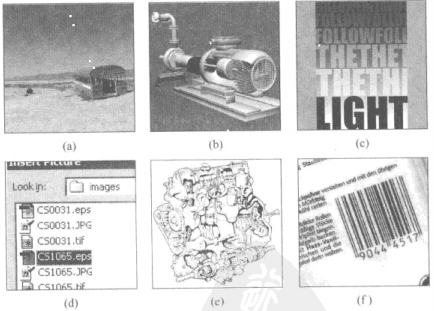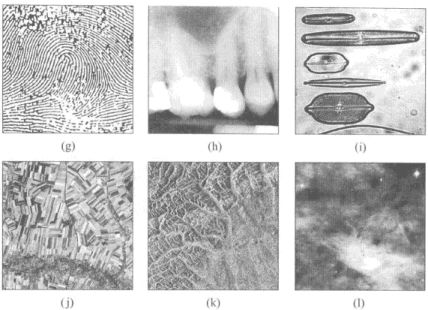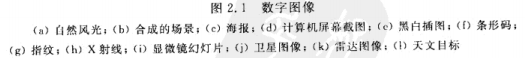## 2.2 图像获取

### 2.2.1 针孔照相机模型

#### 透视变换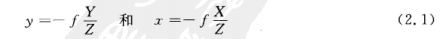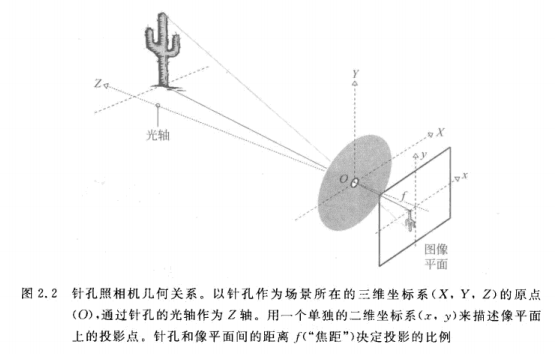### 2.2.3 数字化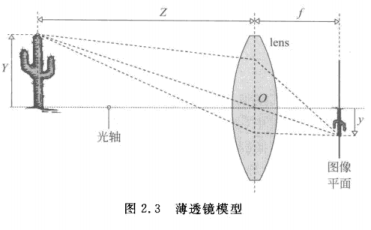1.连接的光线分布必须被空间采样。
2.步骤1的采样结果必须在时间域被采样，以创建一幅图像。
3.最后，采样的值必须被量化到有限范围的整数，这样它们才能在计算机中被表示。

#### 步骤1：空间采样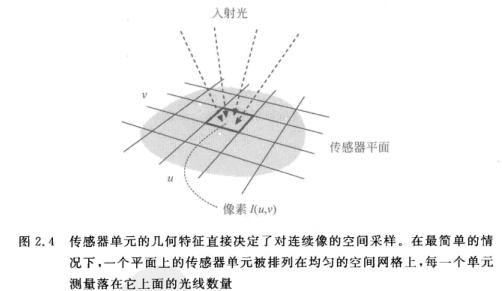#### 步骤3：量化像素值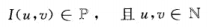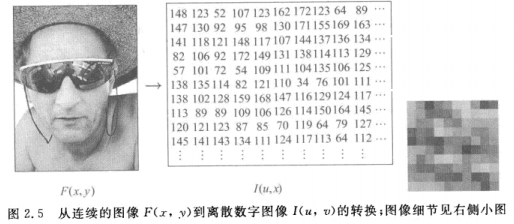### 2.2.5 图像坐标系统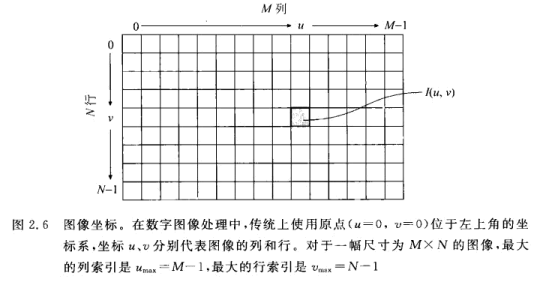### 2.2.6 像素值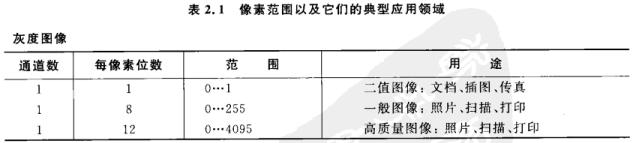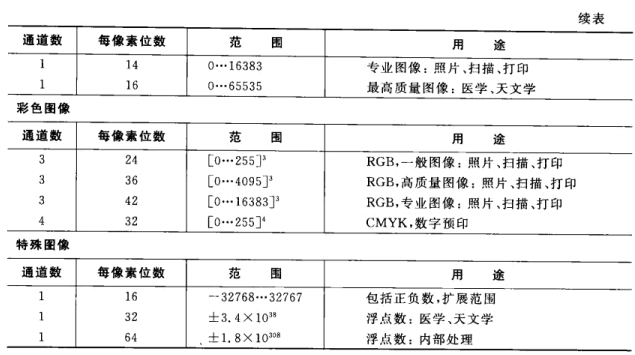# 第三章 ImageJ

## 3.2 ImageJ综述

ImageJ是本书所采用的软件，它是以上讨论的两类工具的结合。它提供了一套现成的工具，用于图像的查看和交互操作，同时也可以通过应用某种“真正的”编程语言编写新的软件组件来得到扩展。ImageJ完全采用Java语言编写，因此它与平台无关，可以不加修改地运行在Windows、MasOS或者Linux操作系统上。Java的动态执行模型允许将新的模块（“插件”）作为独立的Java代码段来编写，这些代码甚至不需要重新启动ImageJ就可以被编码、加载和在运行的系统中执行，这使得ImageJ成为开发和测试新的图像处理技术和算法的理想平台。由于Java语言作为许多工程课程的首选语言已经非常流行，所以通常学生不需要花费大量的时间去学习另一种编程语言就能够非常轻松地使用ImageJ。同时，ImageJ可免费获取，不论学生、教师还是从业人员都可以合法地在任何计算机上安装使用它，而不必购买许可。因此，ImageJ是一个进行数字图像处理教学和自我训练的理想平台，同时它也在全球范围内的许多实验室里被用于正式的研究和应用程序开发，特别是在生物学和医学的图像处理中。

ImageJ是由美国国家卫生研究院（NIH）的Wayne Rasband开发的，起初是作为它的前身（只能在Apple Macintosh平台下使用的）NIH-Image的替代品。ImageJ的当前版本、更新、文档、全部源代码、测试图像以及不断增加的第三方插件集都可以在ImageJ的网站下载。软件的安装很简单，具体的操作指南可以从网站、Werner Bailer的编程教程以及本书的附录C中获得。

### 3.2.1 关键特征

• 一系列交互式即用工具，用于创建、显示、编辑、处理、分析、加载和存储图像，且支持多种常见文件格式，同时支持16位整型、32位浮点型的图像和图像序列。
• 一种简单的插件机制，通过编写Java代码段（通常较小）来扩展ImageJ的核心功能。本书中所有的代码示例都是基于这种插件的。
• 一种宏语言以及相应的解释器，可以简单地通过合并已有的函数来实现较大的处理模块，不需要任何Java的知识。本书中没有使用宏，但可以在ImageJ的在线文档中找到宏的详细说明。

### 3.2.2 交互式工具

ImageJ启动后首先打开的是主窗口（图3.1），其中包括下列菜单项。

• File: 打开、保存和创建新的图像；
• Edit: 编辑和绘制图像；
• Image: 修改和转换图像、几何操作；
• Process: 图像处理，包括点运算、滤波器以及多幅图像间的算法操作；
• Analyze: 对图像数据进行统计测量、直方图和特殊显示格式；
• Plugin: 编辑、编译、执行和管理用户定义的插件。
• 当前版本的ImageJ可以打开多种常见格式的图像，包括TIFF（仅支持未压缩的）、JPEG、GIF、PNG和BMP，同样也支持医学和天文图像处理中常用的DICOM和FITS格式。与大多数图像编辑程序相同，所有的交互式操作都被应用于当前操作的图像，即由用户选中的图像。ImageJ还提供了一种简单的（单步）“撤销”机制，可以从用户定义的插件的操作结果中恢复。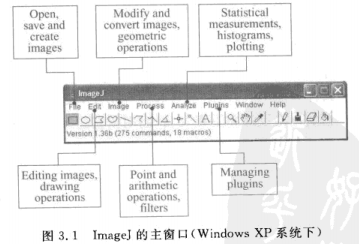### 3.2.3 Image插件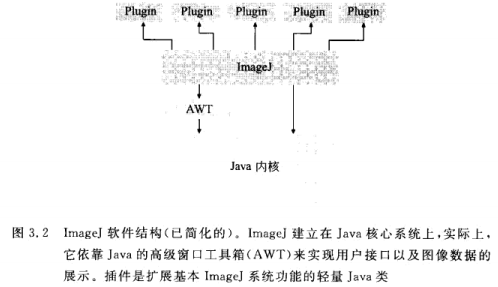• PlugIn： 启动插件时不需要打开一幅图像；
• PlugInFilter：启动插件时当前操作图像被传递给插件；
在本书的所有示例中，我们几乎全部采用第二种类型（PlugInFilter）来实现图像处理操作。接口规范要求所有PlugInFilter类型的插件必须至少实现两种方法：setup()和run()，以下是方法的原型：
int setup(String arg,ImagePlus im)


void run(ImageProcessor ip)


### 3.2.4 第一个例子：图像取反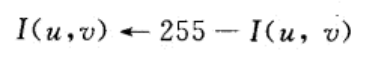#### setup()方法

package com.myplugin;

import ij.ImagePlus;
import ij.plugin.filter.PlugInFilter;
import ij.process.ImageProcessor;

public class My_Inverter implements PlugInFilter{

@Override
public void run(ImageProcessor ip) {
int w = ip.getWidth();
int h = ip.getHeight();

//在图像所有坐标中循环
for (int u = 0; u < w; u++) {
for (int v = 0; v < h; v++) {
int p = ip.getPixel(u, v);
ip.putPixel(u, v, 255-p);
}

}
}

@Override
public int setup(String arg0, ImagePlus arg1) {
return DOES_8G;
}
}


#### run()方法

int getPixel(int u,int v)


void putPixel(int u,int v,int a)


#### 编辑、编译和执行插件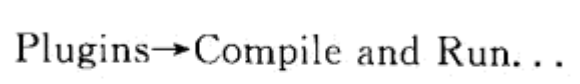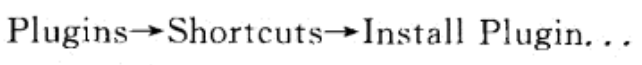# 第四章 直方图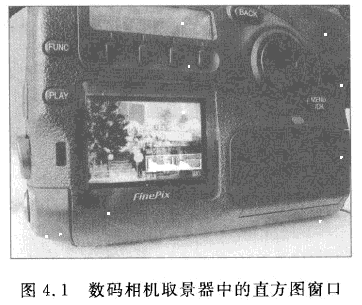## 4.1 什么是直方图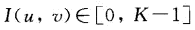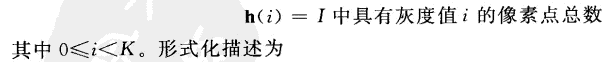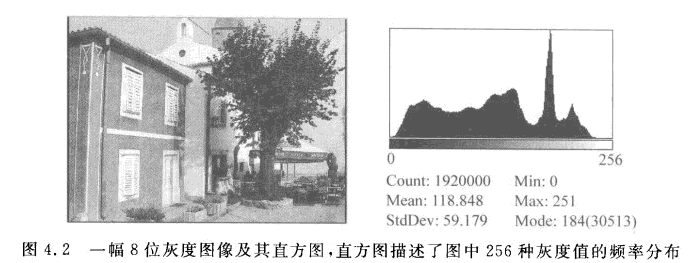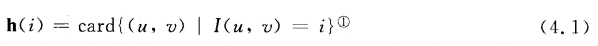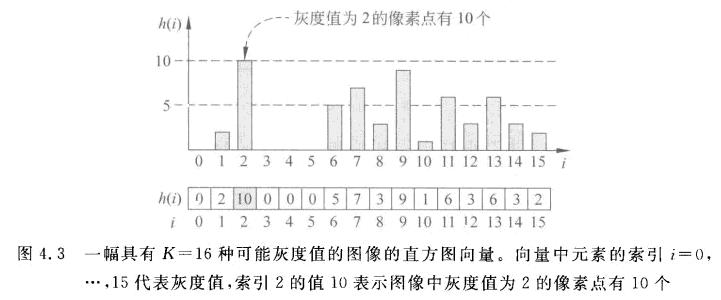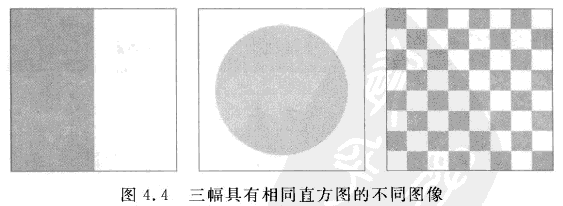## 4.2 解读直方图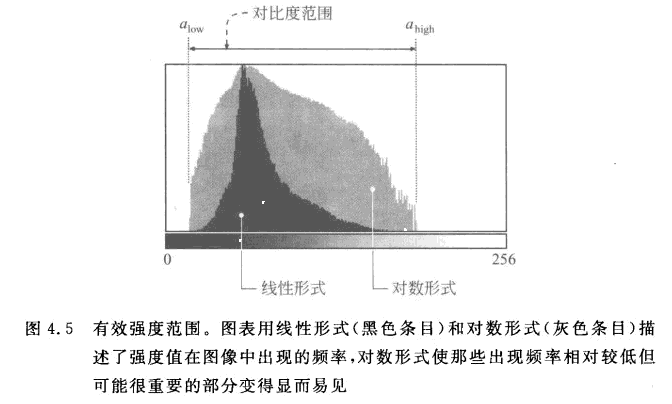### 4.2.1 图像获取

#### 曝光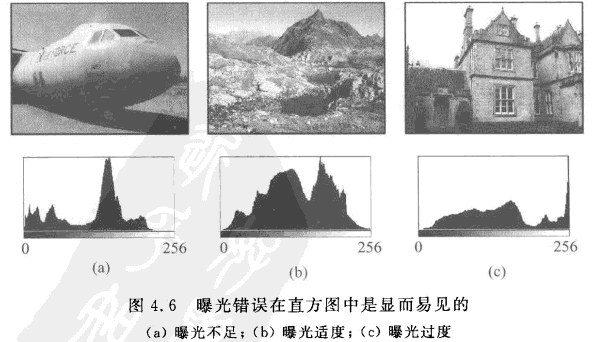#### 对比度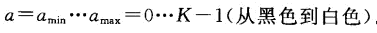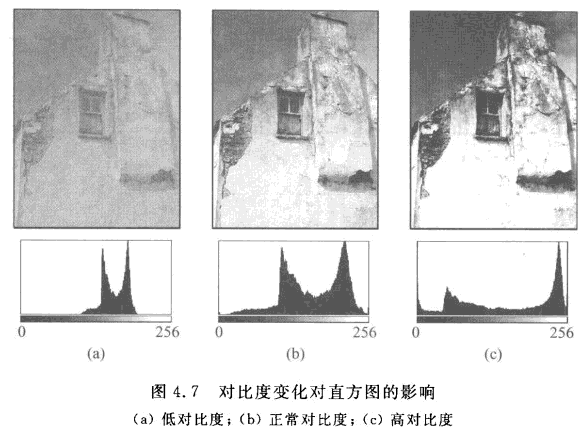#### 动态范围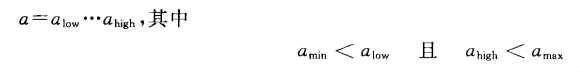## 几种常用的图像处理函数库

2016-10-07 23:27:19 Real_Myth 阅读数 6138
• ###### Linux系统目录及必备命令学习

通过本课程的学习，大家可以从懵懂到熟悉，从熟悉到熟练，能够在企业中熟练运用，同时在学习的过程中有任何不明白的地方，都可以向我咨询，我会积极帮助大家解决问题。

217人学习 吴光科
免费试看

from:http://www.wtoutiao.com/p/18a4MqP.html

OpenCV

OpenCV的全称是：Open Source Computer Vision Library。OpenCV是一个基于（开源）发行的跨平台计算机视觉库，可以运行在Linux、Windows和Mac OS操作系统上。它轻量级而且高效——由一系列 C 函数和少量 C++ 类构成，同时提供了Python、Ruby、MATLAB等语言的接口，实现了图像处理和计算机视觉方面的很多通用算法。

OpenCV用C++语言编写，它的主要接口也是C++语言，但是依然保留了大量的C语言接口。该库也有大量的Python, Java and MATLAB/OCTAVE (版本2.5)的接口。这些语言的API接口函数可以通过在线文档获得。如今也提供对于C#,Ch, Ruby的支持。

CxImage

CxImage类库是一个优秀的图像操作类库。它可以快捷地存取、显示、转换各种图像。有的读者可能说，有那么多优秀的图形库，如OpenIL,FreeImage,PaintLib等等。

CxImage类库是一个优秀的图像操作类库。它可以快捷地存取、显示、转换各种图像。有的读者可能说，有那么多优秀的图形库，如OpenIL,FreeImage,PaintLib等等，它们可谓是功能强大，齐全，没必要用其它的类库。但我要说，这些类库基本上没有免费的，使用这些类库，你要被这样那样的许可协议所束缚。在这点上，CxImage类库是完全免费的。另外，在使用上述类库时，你会遇到重重麻烦。因为它们大部分是平台无关的，且用C语言写成，有的还夹杂着基本的C++ wrapper和成堆德编译选项的声明需要你去处理。而CxImage类库在这方面做得很好。还有让我最看好的，就是作者完全公开了源代码。相对于那些封装好的图形库和GDI+来说，这一点使我们可以进一步学习各种编解码技术，而不再浮于各种技术的表面。

CImg

CImg 库是一个免费、开源的图像处理C++库，名称原意是 Cool Image，正如其名，CImg是一个非常优秀、功能强大、代码简洁、使用方便的C++ 图像处理库。它不仅非常适合科学家、研究生做科研时使用，也适合在工业应用工程开发中使用，更适合的是，对于有志于开发简洁、高效、功能强大的图像处理库的人而言，CImg的源码是不可多得的学习和参考资料。

FreeImage

FreeImage  是一款免费的、开源的、跨平台（Windows 、Linux 和Mac OS X ）的，支持20 多种图像类型的（如BMP 、JPEG 、GIF 、PNG 、TIFF 等）图像处理库。其最大优点就是采用插件驱动型架构，具有快速、灵活、简单易用的特点，得到了广泛使用。

FreeImage 的主要功能有多格式位图的读写；方便的位图信息获取；位深度转换；位图页面访问；基本的几何变换和点处理；通道合并与混合等。FreeImage 暂时不支持矢量图形和高级图像处理，位图绘制需要由用户来完成。

FreeImage 中的所有函数都以FreeImage_ 开头，如图像文件的读写函数分别为FreeImage_Load 和FreeImage_Save 。FIBITMAP 数据结构保存着位图信息和像素数据，是FreeImage 的核心。

C语言的体系，大量使用指针运算速度可以保证，内含先进的多种插值算法。另外独有的支持meta exif信息的读取。该库最大的特点就是比较简练，只把重点放在对各种格式图像的读取写入支持上，没有显示部分，实际编程的时候还是需要调用API函数进行显示。

## 基于Web的图像处理

2016-01-25 15:28:02 u014136513 阅读数 1435
• ###### Linux系统目录及必备命令学习

通过本课程的学习，大家可以从懵懂到熟悉，从熟悉到熟练，能够在企业中熟练运用，同时在学习的过程中有任何不明白的地方，都可以向我咨询，我会积极帮助大家解决问题。

217人学习 吴光科
免费试看

l  基于Web的图像处理允许用户使用分布式数据，即无论数据存放在何处，只要通过网络或者web能够访问即可。数据可能在桌面端、局域网或者世界上某个角落的服务器上。

l  可以使用Esri发布的地理地图或者其他资源来展示数据，可以使用矢量图层。能够使用目录例如Jagwire管理数据。

1 Web图像处理应用框架

2 获取数据服务

3 植被分析

4 数据目录

5 使用ESE进行图像分类